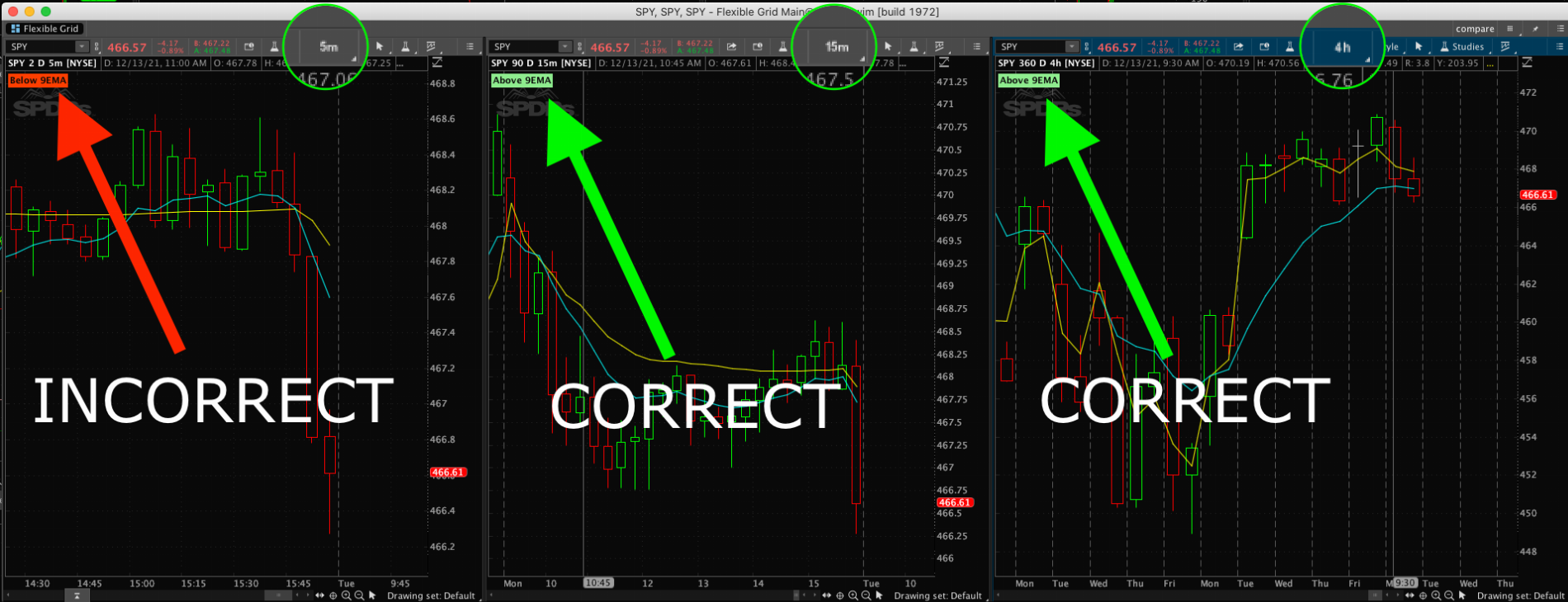# 4 hour label on 5 minute timeframe

#### lucius

##### New member
I made a label to display when 60% of open & close is above or below the 9 EMA in the 4 hour period.
It appears to work as expected for anything at or above the 15 minute time frame, time frames at 5 minute or below, it shows the incorrect value.
Please tell me what I am doing wrong.

Code:
``````input rangePercentValue = .60;
input period = aggregationPeriod.FOUR_HOURS;
input avgType = averageType.EXPONENTIAL;
input length = 9;
input priceClose = close;

def EMA = MovingAverage(avgType, close(period = period), length);

def greenCandleRange = close - open;
def redCandleRange = open - close;

def greenCandleRangeUp = close - (rangePercentValue * greenCandleRange);
def redCandleRangeDown = open - (rangePercentValue * redCandleRange);

#moveUp
def greenAboveEMA = greenCandleRangeUp > EMA;
def redAboveEMA = redCandleRangeDown > EMA;
def moveUp = greenAboveEMA or redAboveEMA;

#moveDown
def greenBelowEMA = greenCandleRangeUp < EMA;
def redBelowEMA = redCandleRangeDown < EMA;
def moveDown = greenBelowEMA or redBelowEMA;

AddLabel(yes, if moveUp then "Above 9EMA" else if moveDown then "Below 9EMA" else "", if moveUp then Color.LIGHT_GREEN else if moveDown then Color.LIGHT_RED else Color.CURRENT );``````I made a label to display when 60% of open & close is above or below the 9 EMA in the 4 hour period.
It appears to work as expected for anything at or above the 15 minute time frame, time frames at 5 minute or below, it shows the incorrect value.
Please tell me what I am doing wrong.

Code:
``````input rangePercentValue = .60;
input period = aggregationPeriod.FOUR_HOURS;
input avgType = averageType.EXPONENTIAL;
input length = 9;
input priceClose = close;

def EMA = MovingAverage(avgType, close(period = period), length);

def greenCandleRange = close - open;
def redCandleRange = open - close;

def greenCandleRangeUp = close - (rangePercentValue * greenCandleRange);
def redCandleRangeDown = open - (rangePercentValue * redCandleRange);

#moveUp
def greenAboveEMA = greenCandleRangeUp > EMA;
def redAboveEMA = redCandleRangeDown > EMA;
def moveUp = greenAboveEMA or redAboveEMA;

#moveDown
def greenBelowEMA = greenCandleRangeUp < EMA;
def redBelowEMA = redCandleRangeDown < EMA;
def moveDown = greenBelowEMA or redBelowEMA;

AddLabel(yes, if moveUp then "Above 9EMA" else if moveDown then "Below 9EMA" else "", if moveUp then Color.LIGHT_GREEN else if moveDown then Color.LIGHT_RED else Color.CURRENT );``````As the 4 hour timeframe opens with a 9am bar, it might create different values than other timeframes. As Ondemand is not available, I could not test your specific issue.

However, I would suggest that you revise your code as follows to ensure that the values on the 4 hour are what is being used on any chart timeframe using this script.

Ruby:
``````input rangePercentValue = .60;
input period = AggregationPeriod.FOUR_HOURS;
input avgType = AverageType.EXPONENTIAL;
input length = 9;
input priceClose = close;

def EMA = MovingAverage(avgType, close(period = period), length);

def greenCandleRange = close(period = period) - open(period = period);
def redCandleRange = open(period = period) - close(period = period);

def greenCandleRangeUp = close(period = period) - (rangePercentValue * greenCandleRange);
def redCandleRangeDown = open(period = period) - (rangePercentValue * redCandleRange);

#moveUp
def greenAboveEMA = greenCandleRangeUp > EMA;
def redAboveEMA = redCandleRangeDown > EMA;
def moveUp = greenAboveEMA or redAboveEMA;

#moveDown
def greenBelowEMA = greenCandleRangeUp < EMA;
def redBelowEMA = redCandleRangeDown < EMA;
def moveDown = greenBelowEMA or redBelowEMA;

AddLabel(yes, if moveUp then "Above 9EMA" else if moveDown then "Below 9EMA" else "", if moveUp then Color.LIGHT_GREEN else if moveDown then Color.LIGHT_RED else Color.CURRENT );
AddLabel(1, moveUp + "  " + moveDown, Color.WHITE);``````

•lucius

### Not the exact question you're looking for?

87k+ Posts
111 Online## The Market Trading Game Changer

Join 2,500+ subscribers inside the useThinkScript VIP Membership Club
• Exclusive indicators
• Proven strategies & setups
• Private Discord community
• Exclusive members-only content
• 1 full year of unlimited support

What is useThinkScript?

useThinkScript is the #1 community of stock market investors using indicators and other tools to power their trading strategies. Traders of all skill levels use our forums to learn about scripting and indicators, help each other, and discover new ways to gain an edge in the markets.

How do I get started?

We get it. Our forum can be intimidating, if not overwhelming. With thousands of topics, tens of thousands of posts, our community has created an incredibly deep knowledge base for stock traders. No one can ever exhaust every resource provided on our site.

If you are new, or just looking for guidance, here are some helpful links to get you started.

What are the benefits of VIP Membership?